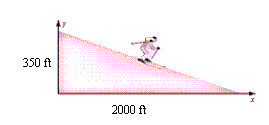Name:    Quiz B.4-B.5

Multiple Choice
Identify the choice that best completes the statement or answers the question.

Graph the line with the given slope that passes through the given point.

1.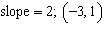a.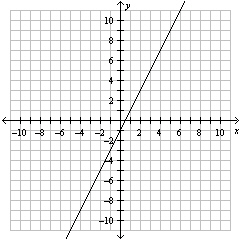c.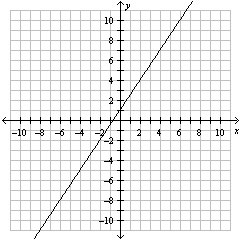b.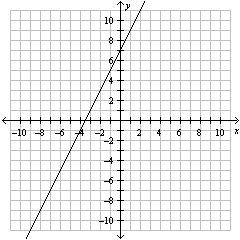d.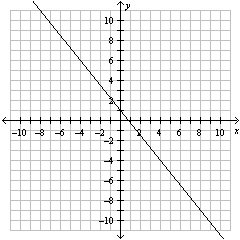2.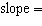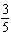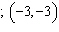a.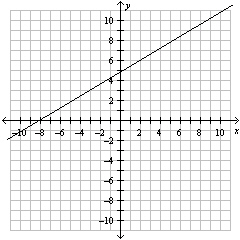c.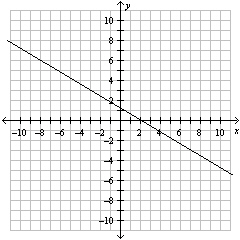b.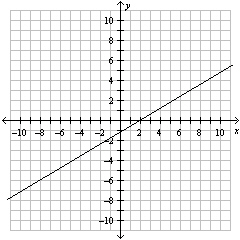d.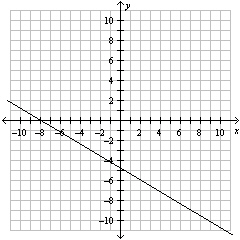3.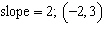a.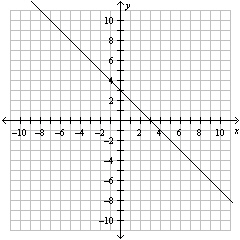c.b.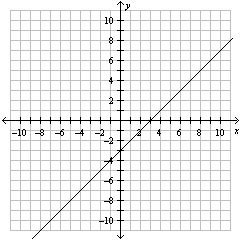d.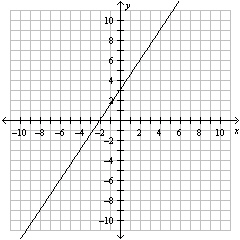4.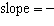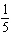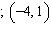a.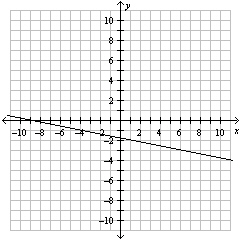c.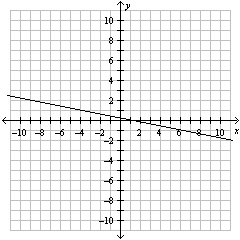b.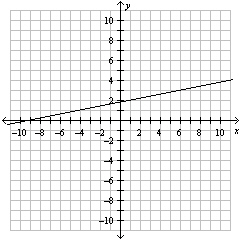d.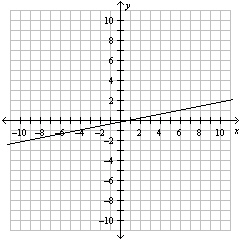5.

What point is 2 units right and 4 units up from (7,3)?
 a. (5, 7) c. (–5, –1) b. (9, 7) d. (–9, 7)

Find the slope and y-intercept of the graph of the linear function.

6.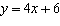a.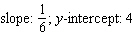c.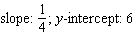b.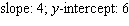d.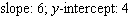7.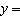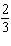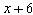a. Slope: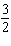; y-intercept: 6 c. Slope:; y-intercept: 6 b. Slope: 4; y-intercept:d. Slope: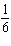; y-intercept:8.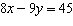a.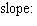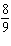: y-intercept: –5 c.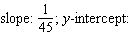b.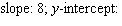d.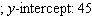9.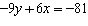a.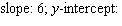c.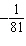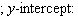b.; y-intercept: –81 d.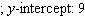Graph the linear function using slope-intercept form.

10.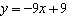a.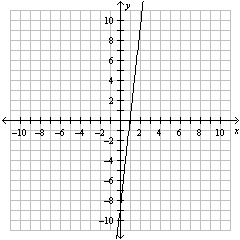c.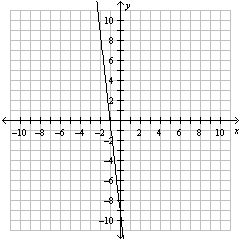b.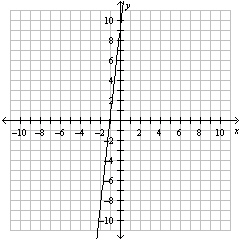d.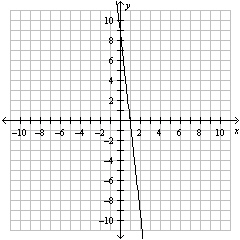11.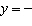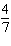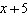a.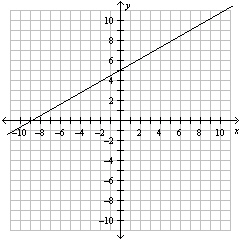c.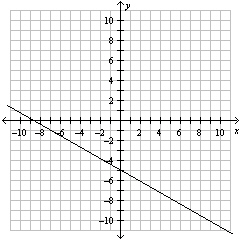b.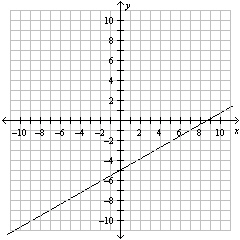d.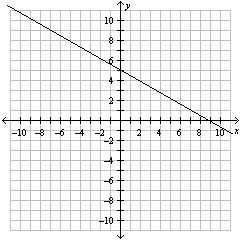12.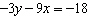a.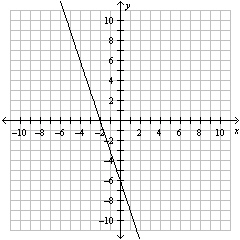c.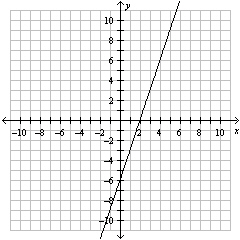b.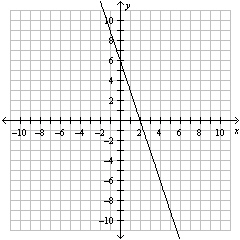d.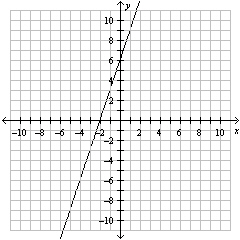Find the slope of the line.

1.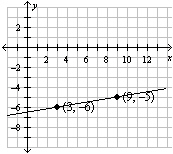2.

What is the slope of the ski hill?